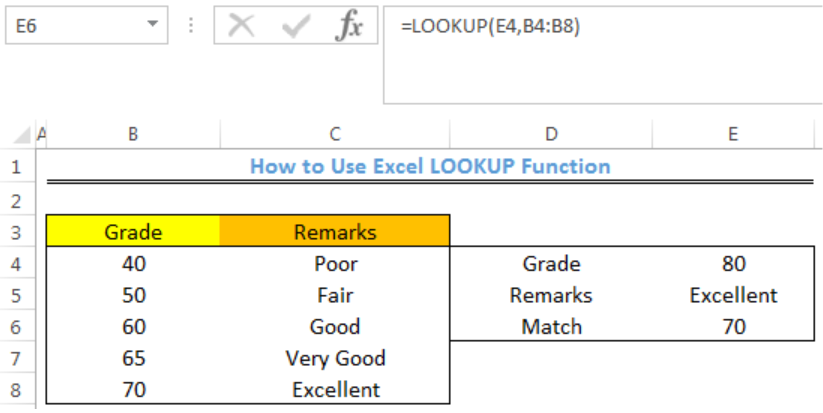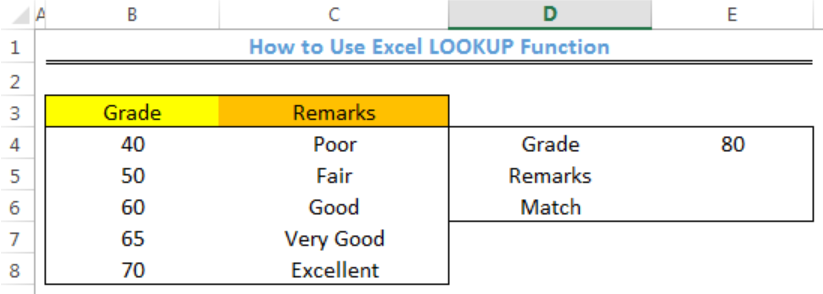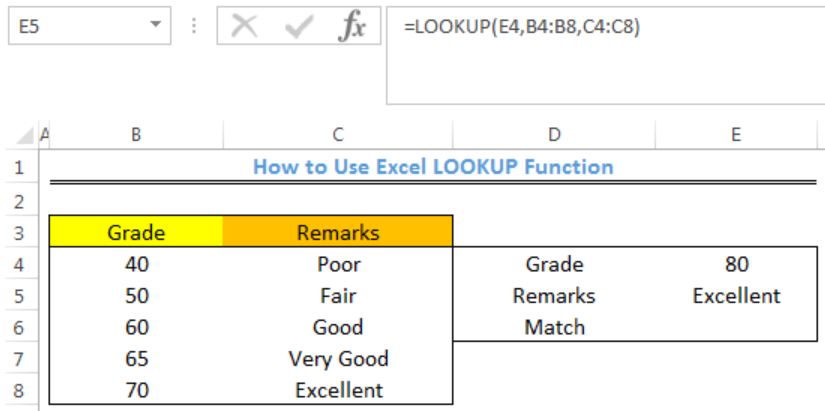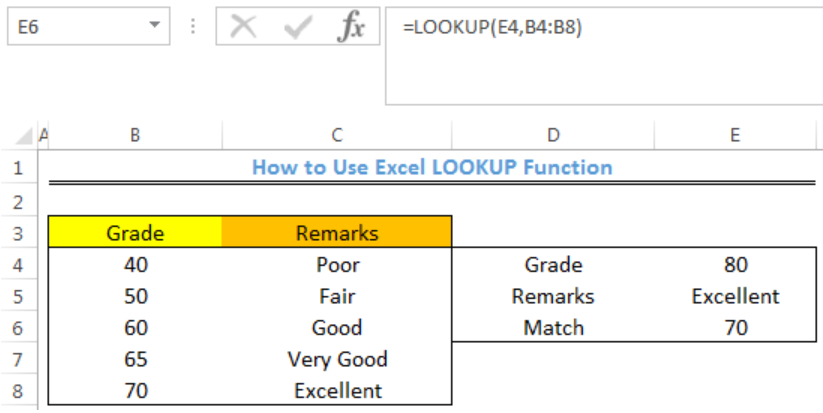Get instant live expert help with Excel or Google Sheets“My Excelchat expert helped me in less than 20 minutes, saving me what would have been 5 hours of work!”

#### Post your problem and you’ll get expert help in seconds.

Your message must be at least 40 characters
Our professional experts are available now. Your privacy is guaranteed.

# Pro Tips on Using the LOOKUP Function in Excel

We can use the Excel LOOKUP function to get an approximate match of the lookup value in a one-column or one-row range and return the corresponding value from another one-column or one-row range. The steps below will walk through the process.Figure 1- How to Use the LOOKUP Function in Excel

## Syntax

`=LOOKUP(lookup_value, lookup_vector, [result_vector])`

• Lookup_value: This is the value to search for
• Lookup_vector: This is the one-row, or one-column range to search for the lookup_value
• Result_vector – [optional]: The one-row, or one-column range of results

## Formula

Remarks: `=LOOKUP(E4,B4:B8,C4:C8)`

Match: `=LOOKUP(E4,B4:B8)`

## Setting up the Data

We will use the LOOKUP FUNCTION to lookup the range and obtain the Remarks for a Grade of 80 and the Match in figure 2.

• The Grades will be entered into Column B
• Column C contains the Remarks
• The results of the LOOKUP FUNCTION will be returned in Column EFigure 2 – Setting up the Data

## LOOKUP Remarks

• We will click on Cell E5
• We will insert the formula below into Cell E5
`=LOOKUP(E4,B4:B8,C4:C8)`
• We will press the enter keyFigure 3- Lookup result for Remarks

## LOOKUP Match

The lookup function can return the value of a cell once it has located its position. However, when it doesn’t find an exact match for the lookup value, the LOOKUP FUNCTION returns the next smaller number as the result.

• We will click on Cell E6
• We will insert the formula below into Cell E6
`=LOOKUP(E4,B4:B8)`
• We will press the enter keyFigure 4- Lookup result for Grade

## Note

• Lookup function has the vector and array form
• This example describes the vector form of Lookup
• The LOOKUP function assumes that the lookup_vector is sorted in ascending order
• If the lookup_value is greater than every value in lookup_vector, the LOOKUP function matches the last value

If the lookup_value is less than all (i.e. the first) value in lookup_vector, the LOOKUP function returns the #N/A error. “The result_vector must be the same size as the lookup_vector.” The LOOKUP function isn’t case-sensitive.

## Instant Connection to an Expert through our Excelchat Service

Most of the time, the problem you will need to solve will be more complex than a simple application of a formula or function. If you want to save hours of research and frustration, try our live Excelchat service! Our Excel Experts are available 24/7 to answer any Excel question you may have. We guarantee a connection within 30 seconds and a customized solution within 20 minutes.

Solution examplesHi, my VLOOKUP isn't working. No matter what I do it is returning the same result regardless of the sku it is searching for - even if I copy and paste the sky into the sheet the exact same way it is typed in the sheet its searching
Solved by X. H. in 20 minsI can't make VLOOKUP work. I have 2 columns. Column A with a complete 11 digit NDC number, Column B contains a 9 digit NDC with the right 2 digits removed. I'm trying to populate column C with the full 11 digit number from column A based on a partial match with column B.
Solved by M. A. in 17 minsIn cells F6:F13, add a VLOOKUP function that returns the raise recommendationâ??High, Standard, or Lowâ??for each employee by exactly matching the Name in column A in the range you named Evaluations. Be sure to use exact match criteria. In cells G6:G13, add a VLOOKUP function that returns the starting raise percentage based on the ranges in the Standard Raise table in cells A16:B19 and the number of years employed in column E.
Solved by X. J. in 20 minsUse the HLOOKUP function in cell H11 to calculate freight cost based on the shipping class and state to which the shipment is going. Freight Cost can be referenced on the "Freight Cost" lookup table (range E5:N8). Be sure to use appropriate relative and absolute cell references. Hint: You will need to use a function within the HLOOKUP function to determine the appropriate row.
Solved by X. C. in 12 minsi want to hide a column but still use it in formula. is this even possible?
Solved by X. E. in 11 mins